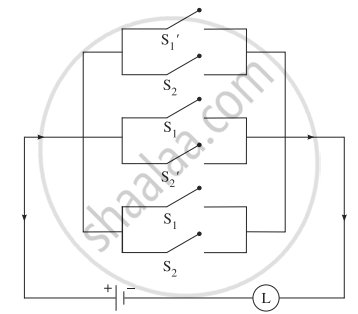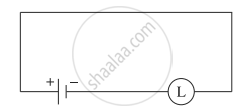# Simplify the following so that the new circuit. - Mathematics and Statistics

Sum

Simplify the following so that the new circuit.#### Solution

Let p: the switch S1 is closed
q: the switch S2 is closed
∼p: the switch S1′ is closed or the switch S1 is open
∼q: the switch S2′ is closed or the switch S2 is open.
Then the symbolic form of the given switching circuit is:
(∼p ∨ q) ∨ (p ∨ ∼q) ∨ (p ∨ q)
Using the laws of logic, we have,
(∼p ∨ q) ∨ (p ∨ ∼q) ∨ (p ∨ q)
≡ (∼p ∨ q ∨ p ∨ q) ∨ (p ∨q)
≡ [(∼p ∨ p) ∨ (q ∨ q)] ∨ (p ∨ q) ......(By Commutative Law)
≡ (T ∨ T) ∨ (p ∨ q) ..........(By Complement Law)
≡ T ∨ (p ∨ q) .......(By Identity Law)
≡ T .......(By Identity Law)
∴ the current always flows whether the switches are open or closed. So, it is not necessary to use any switch in the circuit.
∴ the simplified form of the given circuit is:Concept: Application of Logic to Switching Circuits
Is there an error in this question or solution?

#### APPEARS IN

Balbharati Mathematics and Statistics 1 (Arts and Science) 12th Standard HSC Maharashtra State Board
Chapter 1 Mathematical Logic
Miscellaneous Exercise 1 | Q 16 | Page 35